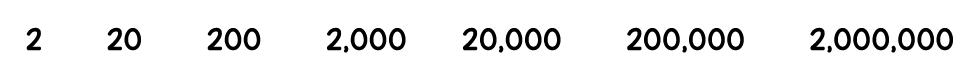# Lesson 3: Digits to the Left and Right

## Getting Started

What would you like 10 times more of? For example, if a friend gave you one dollar, would you prefer to have ten dollars? Or would you like to have 10 times more minutes to play video games or read or be with friends?

Is there anything you'd like 1/10 of? For example, would you like 1/10 the amount of schoolwork or 1/10 the number of chores? Keep in mind that a fraction is less than a whole. So having 1/10 of your chores would mean having fewer chores.

When we're very young, we're taught to count by ones, but often, it's faster to count by tens, and because our number system is based on the number ten, counting by tens is easy.

Think about these numbers, what they mean, and how they compare to one another. For example, how does 2 compare to 20? And how does 200,000 compare to 2,000,000?When you first learned about place value, you probably worked with base-ten blocks. These blocks include unit blocks, ten rods, hundred flats, and thousand cubes. You probably remember that 10 unit blocks equal 1 ten rod, that 10 ten rods equal 1 hundred flat, and that 10 hundred flats equal 1 thousand cube.During this lesson, you're going to extend your understanding of basic place value to explore patterns when moving from one place value to another, going either left or right.

### Stuff You Need

• calculator
• Interactive Notebook

• How does place value work?
• How do you read and write decimal numbers?

### Things to Know

• Decimal number: a number that has a decimal point
• Decimal point: the most important part of a decimal number that shows where numbers begin getting larger than 1 (to the left of the decimal point) and where they begin getting smaller than 1 (to the right of the decimal point)
• The digits to the left of the decimal point are whole numbers.
• The digit to the right of a decimal point is in the tenths place, and the digit to the right of the tenths place is in the hundredths place.
• A digit in one place represents 10 times as much as it represents in the place to its right and 1/10 of what it represents in the place to its left.

### Skills

• Recognize that in a multi-digit number, a digit in one place represents 10 times as much as it represents in the place to its right and 1/10 of what it represents in the place to its left

### Introducing the Lesson

As your child builds his mastery of large numbers and decimals, it's critical that he understands that a digit in one place represents 10 times as much as it represents in the place to its right and 1/10 of what it represents in the place to its left. For example, 200 is 10 times 20, and 200 is 1/10 of 2,000. Your child will work on solidifying his understanding during this lesson and will use this understanding in subsequent lessons. This lesson briefly reviews decimal numbers, focusing on moving the decimal point when multiplying or dividing by 10. Your child will review decimals more thoroughly during Lesson 4.